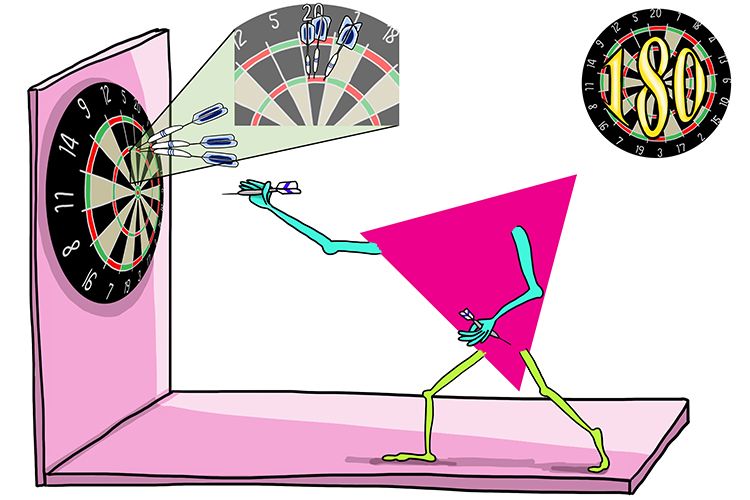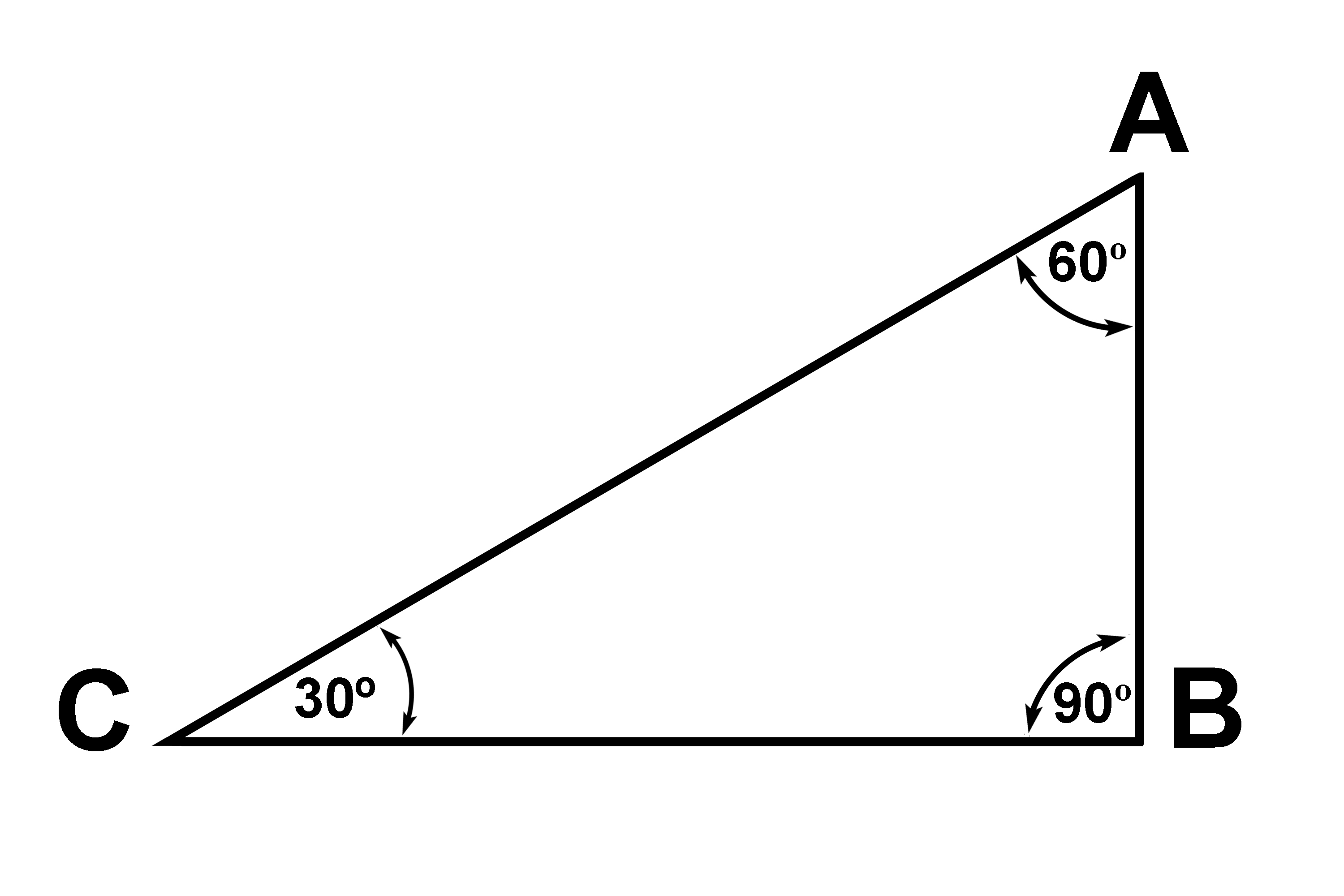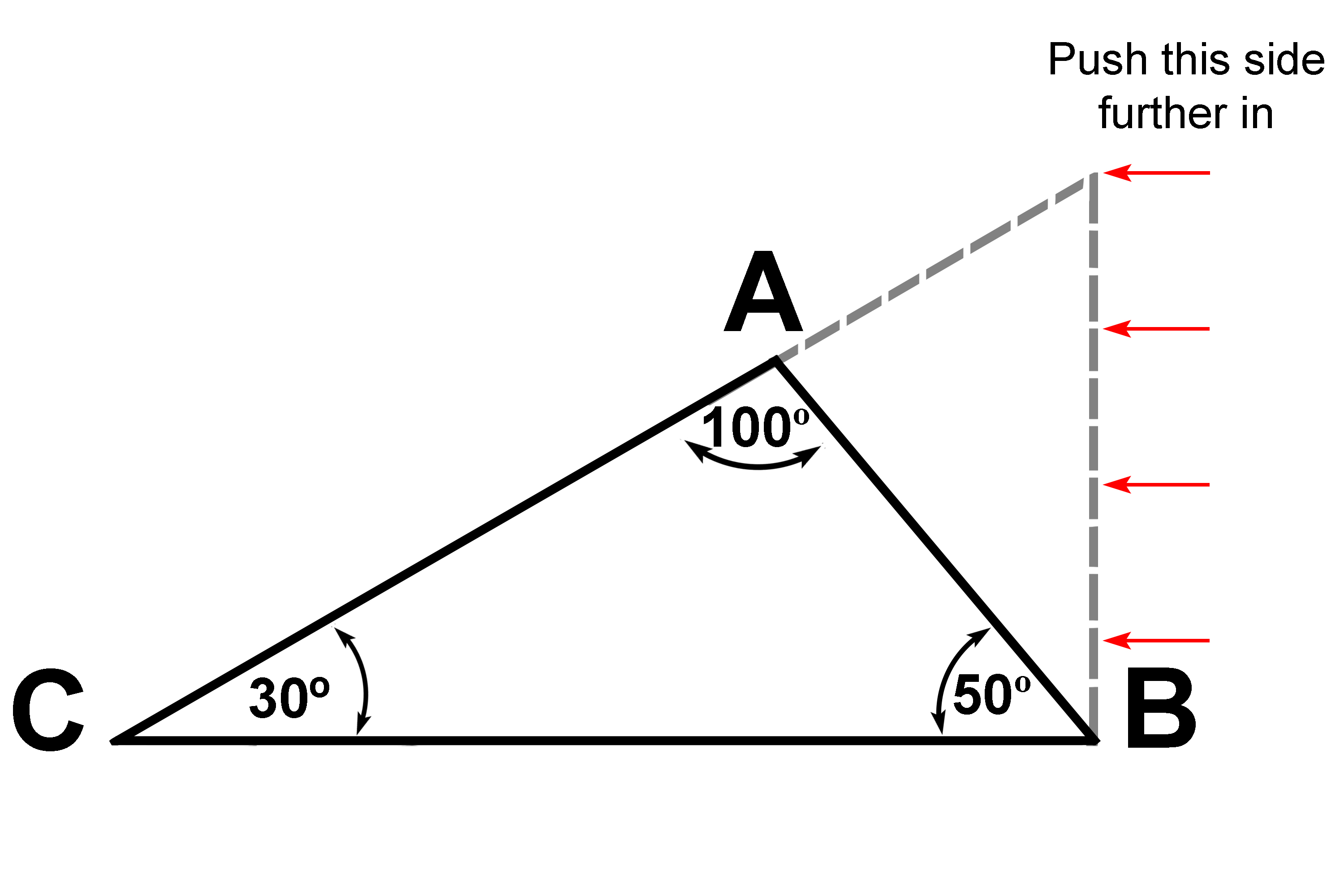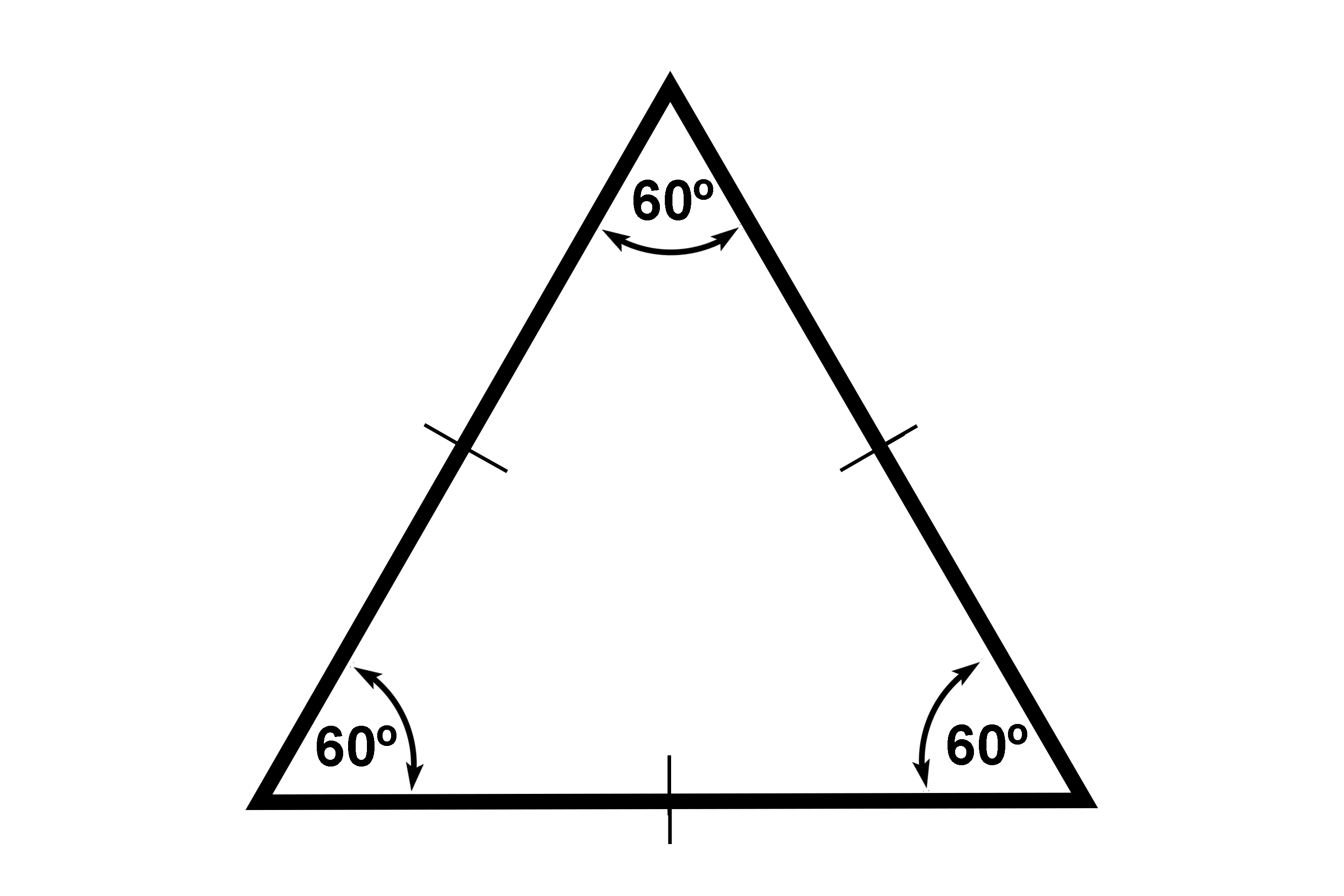# The total interior angles of a triangle = 180°The triangle scored three treble twenties in her darts game which gave a combined score of 180.

The total internal angles of a triangle = 180°

Example 160°+90°+30°=180°

The interior angles of a triangle add up to 180°

Example 2

All we have done in this example is push the one side (A-B) of the triangle further in.100°+50°+30°=180°

Example 3

An equilateral triangle will have 3 equal internal angles and would be

180^circ/3=60^circ

Each internal angle is 60°.1 2 3 60° + 60° + 60° = 180°

But the interior angles would still add up to 180°.